# Plus Two Physics Chapter Wise Questions and Answers Chapter 11 Dual Nature of Radiation and Matter

Students can Download Chapter 11 Dual Nature of Radiation and Matter Questions and Answers, Plus Two Physics Chapter Wise Questions and Answers helps you to revise the complete Kerala State Syllabus and score more marks in your examinations.

## Kerala Plus Two Physics Chapter Wise Questions and Answers Chapter 11 Dual Nature of Radiation and Matter

### Plus Two Physics Dual Nature of Radiation and Matter NCERT Text Book Questions and Answers

Question 1.
Find the

1. Maximum frequency, and
2. Minimum wavelength of X-rays produced by 30 kV electrons.

Given Vo = 30 kV = 30 × 103 V
vmax = ?
λmax = ?
1. Since kmax = eVo
So hvmax = eVo
or= 7.24 × 1018 Hz

2.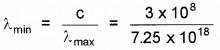= 0.041 × 10-9
or λmin = 0.041 nm.Question 2.
The work function of caesium metal is 2.14 eV. When light of frequency 6 × 1014Hz is incident on the metal surface, photoemission of electrons occurs. What is the

1. maximum kinetic energy of the emitted electrons,
2. maximum speed of the emitted photoelectrons?

Given W0 = 2.14 eV
= 2.14 × 1.6 × 10-19 J
= 3.424 × 10-19J
v = 6 × 1014 Hz

1. Kmax = hv – W0
= 6.62 × 10-34 × 6 × 1014 – 3.424 × 1019
= 3.972 × 10-19 – 3.424 × 10-19
= 0.54 × 10-19 J.

2. Since eV0 =kmax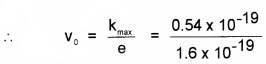or v0 = 0.34 V
Since $$\frac{1}{2}$$ mV2max = Kmax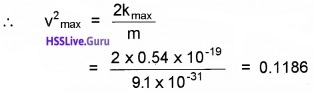or vmax = 0.344 × 106 ms-1 = 344 kms-1Question 3.
The photoelectronic cut-off voltage in a certain experiment is 1.5 V. What is the maximum kinetic energy of photoelectrons emitted?
Given V0 = 1.5 V
Since kmax = eV0
= 1.5 eV
= 1.5 × 1.6 × 10-19 J = 2.4 × 10-19 J.

Question 4.
In an experiment on photoelectric effect, the slope of the cutoff voltage versus frequency of incident light is found to be 4.12 × 10-15Vs. Calculate the value of Planck’s constant.
Given, slope of graph = 4.12 × 10-15 Vs
since, slope of graph = $$\frac{h}{e}$$
∴ h = e × slope of graph
= 1.6 × 10-19 × 4.12 × 10-15 = 6.59 × 10-34 Js.Question 5.
The threshold frequency fora certain metal is 3.3 × 1014 Hz. If light of frequency 8.2 × 1014 Hz is incident on the metal, predict the cutoff voltage for the photoelectric emission.
Given v0 = 3.3 × 1014Hz
v = 8.2 × 1014Hz
Since eV0 = hv – hv0
So V0 = $$\frac{h}{e}$$ (v – v0)
$$=\frac{6.62 \times 10^{-34}}{1.6 \times 10^{-19}}$$ × (8.2 × 1014 – 3.3 × 1014)
= 4.14 × 10-15 × 4.9 × 1014
= 2.02 V = 2.0 V

Question 6.
Light of frequency 7.21 × 1014Hz is incident on a metal surface. Electrons with a maximum speed of 6.0 × 105m/s are ejected from the surface. What is the threshold frequency for photoemission of electrons?
Given v = 7.21 × 1014Hz
umax = 6.0 × 1014ms-1
v0 = ?
Since Kmax = hv – hv0
∴ v0 = v – Kmax### Plus Two Physics Dual Nature of Radiation and Matter One Mark Questions and Answers

Question 1.
Find out the wrong statement
(i) As frequency increases photo current increases
(ii) As frequency increase KE increases
(iii) As frequency increase velocity of electrons increases
(iv) As frequency increase stopping potential increases
(v) As frequency is below a certain value photo electrons are not emitted.
(i) As frequency increases photo current increases.Question 2.
Read the following statements and write whether true or false.

1. During photo electric effect photon share its energy with a group of electrons.
2. Intensity is directly proportional to square of amplitude

1. False
2. True

Question 3.
In photoelectric emission the number of photoelectrons emitted per second depends on
(a) wavelength of incident light
(b) frequency of incident light
(c) intensity of incident light
(d) work function of the material
(c) intensity of incident lightQuestion 4.
What is de-Broglie wave?
The wave associated with material particle is called de-Broglie wave.

Question 5.
In photoelectric emission process from a metal of work function 1.8 eV, the kinetic energy of most energetic electrons is 0.5 eV. The corresponding stopping potential is
(a) 1.8 V
(b) 1.3 V
(c) 0.5 V
(d) 2.3 V
(c) 0.5 V
Explanation:
The stopping potential Vs is related to the maximum kinetic energy of the emitted electrons Kmax through the relation
Kmax = eVs
0.5 eV = eVs or Vs = 0.5 VQuestion 6.
Name the experiment, which establish the wave nature of moving electrons.
Davisson Germer experiment.

Question 7.
Pick the odd one out of the following,
(a) Interference
(b) Diffraction
(c) Polarization
(d) Photoelectric effect
(d) Photoelectric effect

Question 8.
Find out the wrong statement

1. If two particles have same momentum then they have same de-Broglie wave length.
2. If two particles have same KE the lighter particle has smallerwave length
3. As velocity of a given mass decreases wave-length increases

1. True
2. False
3. TrueQuestion 9.
Pick the odd one out from the following x-rays, visible light, matter waves, radio waves
Matter waves.

Question 10.
If the electrons are accelerated by a potential of 50V, calculate the de-Broglie wavelength of electrons.### Plus Two Physics Dual Nature of Radiation and Matter Two Mark Questions and Answers

Question 1.
Classify the following properties of the waves into de Broglie wave, em wave, and sound wave.

1. Associated with the moving particle.
2. Longitudinal wave
3. Electric field and magnetic field are perpendicular to each other.
4. Can produce photo electric effect.
5. Wave length is inversely proportional to mass of the moving particle.
6. Velocity in vacuum is 3×108 m/s.

1. de-Broglie wave – 1,5
2. Em wave – 3,4,6
3. Sound wave – 2Question 2.
Table given below gives the work function of certain elements.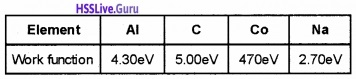Identify the element in which photoelectric effect occurs easily. Justify your answer.
Na – 2.70eV, work function is least.

Question 3.
“Louis De Broglie suggested existence of matter waves based on a hypothesis”

1. What do you mean by matter wave?
2. The objects in our daily life do no exhibit wave like properties. Why?

1. The wave associated with material particle is called matterwave.
2. objects in ourdaily life have large mass. Hence λ is very small (In the order of 10-34 cm) to be neglected.Question 4.
A body of mass 1 Kg is moving with a velocity 1 m/s, a wave is associated with this body

1. Name the wave
2. Can you measure wave length of this wave. Explain?

1. Matterwave
2. No. wave length of matterwave is very small.

### Plus Two Physics Dual Nature of Radiation and Matter Three Mark Questions and Answers

Question 1.
In figure below represents the variation of current with potential fora metal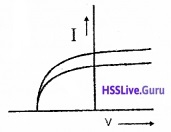1. Identify the law governing it.
2. Even when the potential is zero, there is current. Explain.
3. Current is zero for a particular potential. How does this potential help in determining the velocity of electrons.

1. Laws of photoelectric emission

• For a given frequency of radiation, number of photoelectrons emitted is proportional to the intensity of incident radiation.
• The K.E. of photoelectrons depends on the frequency of incident light but is independent of the light intensity.

2. When radiation falls on metal, photo electrons are emitted with certain velocity even if accelerating potential is zero.

3. At slopping potential (v0), photocurrent is zero.
ie. 1/2 mv2max = ev0Question 2.
A metal whose work function is 2 eV is illuminated by light of wavelength 3 × 10-7 m. Calculate

1. threshold frequency
2. maximum energy of photoelectrons
3. the stopping potential.

F0 = h ν0 – 2eV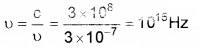1. F0 = h ν02. 1/2mv2 = h(ν – ν0)
= 6.63 × 10-34 (1015 – 4.8 × 1014)
= 3.44 × 10-19J

3. eV0 = h(ν – ν0)Question 3.
The wave nature of electron was experimentally verified by diffraction of electron by Nickel crystal.

1. Name the experiment which establish wave nature of moving electron.
2. An electron and a proton have same kinetic energy which of these particles has shortest de-Broglie wave length?

1. Davisson and Gemner experiment

2.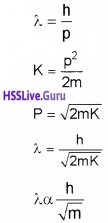Mass of alpha particle is more than that of proton, hence it has shortest wavelength.

Question 4.
“Moving particles of matter shows wave like properties under suitable conditions’’

1. Who put forward this hypothesis?
2. A proton and an electron have been accelerated through same potential. Which one have higher matter wave length. Write the reason

1. De broglie

2. $$\lambda=\frac{\mathrm{h}}{\sqrt{2 \mathrm{meV}}}$$
The mass of proton is higher than electron. Hence wave length of proton is less than electron.Question 5.
Three light beams of same frequency and different intensity I1, I2 and I3 are incident on the same metal. I1 >I2 >I3.

1. Which beam produce maximum photocurrent?
2. Which beam produce electrons of maximum speed and KE?
3. Draw a graph showing variation of photocurrent with intensity in same speed.

1. I3.

2. Frequency is same. Hence electron emitted from the metals will be same.

3.### Plus Two Physics Dual Nature of Radiation and Matter Four Mark Questions and Answers

Question 1.
The graph shows photoelectric current with anode potential.1. The potential at ‘O’ is called

• accelerating potential
• retarding potential
• stopping potential
• saturation potential

2. Why current becomes constant in the region BC?

3.1. Stopping potential
2. The whole electrons emitted from the cathode will reach at anode. Hence current becomes saturation at BC.
3. This graph is not possible. Stopping potential is directly proportional to frequency of incident light, ie. when frequency of incident light increases, stopping potential also increases. Hence we expect a high stopping potential for v2.Question 2.
The magnification of an electron microscope is much larger than that of an optical microscope, because electron beams are used instead of light beams in an electron microscope.

1. Which property of electrons is used in the construction of the electron microscope?
2. Obtain expression for the wavelength of de-Droglie waves associated with an electron accelerated through a potential of V volts.

1. Wave nature.

2. ev = 1/2mv2
mv2 = 2 eV
m2v2 = 2eVm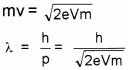Question 3.
The wavelengths of violet and red ends of visible spectrum are 390nm and 760 nm respectively.
1. Evaluate the energy range of the photons of the visible light in electron volts.
2. The work function of four different materials is given in the table below.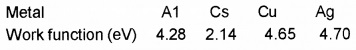Pick out the suitable metal/ metals for the construction of the photo cell which is to operate with visible light.
3. Calculate the threshold frequency of the selected metal/metals.
1. 1.65ev to 3.1ev

2. Cs

3.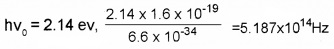Question 4.
“To emit a free electron from a metal surface a minimum amount of energy must be supplied”.

1. It is called……..
2. Give three method to supply energy to a free electron
3. For metal A (tungsten) – work function is 4.52 eV for metal B (thoriated tungsten) it is 2.6 ev, for metallic (oxide coated tungsten) it is 1 eV. Which will you prefer as a good electron emitter and why?

1. Threshold energy
2. Give light energy or heat energy
3. Work function for metallic oxide coated tungsten is small (1 ev.) Hence this material is good electron emitter.

Question 5.
Louis de Broglie argued that electron in circular orbit as proposed by Bohr, must be seen as a Particl wave.

1. From Bohr’s postulate of angular quantization momentum, arrive at an expression for wave length of an orbital electron. (2)
2. Comment on the above result (2)

1.2. Since λ = $$\frac{2 \pi r}{n}$$, length of the first orbit is the de-Broglie wavelength of the orbit.

### Plus Two Physics Dual Nature of Radiation and Matter Five Mark Questions and Answers

Question 1.
Schematic diagram of an experimental set up to study the wave nature of electron is shown below.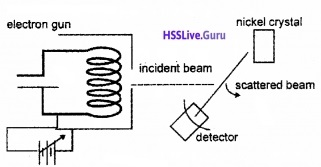1. Identify the experiment.
2. In the experiment the intensity of electron beam is measured for different values of ‘q’. At 54V accelerating potential and q = 50°, a sharp diffraction maximum is obtained. What is the wave length associated with the electron.
3. A particle is moving three times as fast as electron. The ratio of debroglie wavelength of the particle to that electron is 1.813 × 10-14. Calculate the mass of the particle.

1. Davisson and German experiment

2. $$\lambda=\sqrt{\frac{150}{v}}=\sqrt{\frac{150}{54}}=1.66 \mathrm{A}^{0}$$

3.Question 2.
An electron moves under a potential difference of 300V

1. The wave associated with electron is called……….
2. Derive an expression for its wave in terms of charge of particle.
3. Calculate the wavelength of above electron.

1. matter wave

2.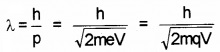3.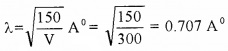Question 3.
Figure below shows a version of Young’s Experiment performed by directing a beam of electrons on double slit. The screen reveals a pattern of bright and dark fringes similar to an interference as pattern produced when a beam of light is used.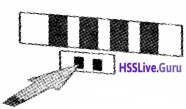1. Which property of electron is revealed in this observation?
2. If the electrons are accelerated by a p.d. of 54V, what is the value of wavelength associated with electrons.
3. In similar experiment if the electron beam is replaced by bullets fired form a gun, no interference pattern is observed. Give reason.

1. Dual nature or wave nature.

2.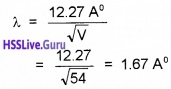3. λ = $$\frac{\mathrm{h}}{\mathrm{mv}}$$ Since the mass of the bullet is very large compared to the mass of electron, the de Broglie wavelength is not considerable.Question 4.
A particles moving with KE 5Mev. Its mass is 1.6 × 10-27 Kg

1. What is the energy of particle in joule?
2. Derive an equation of find De Broglie wave length in terms of KE
3. Calculate De-Broglie wave length of above particle.

1. KE = 5 Mev = 5 × 106 × 1.6 × 10-19J

2.3.Question 5.
Einstein got Nobel Prize in 1921 for his explanation of photoelectric effect.

1. In order to start photoelectric emission, the minimum energy acquired by free electron in the metal is called as…… (1)
2. The minimum energy forthe emission of an electron from metallic surface is given below Na: 2.75eV K: 2.3eV Mo:4.17eV Ni:5.15eV Select the metal which is more photo sensitive. Why? (1)
3. Draw variation of photoelectric current with applied voltage for radiation of intensities I1 and I2 (I1 > I2). Comment on the relatiion between intensity of light and photoelectric current. (2)
4. Does Light from a bulb falling on an iron table emit photoelectron? Justify your answer. (1)

1. Work function/ Threshold energy.

2. K is more photosensitive because it has less work function.

3.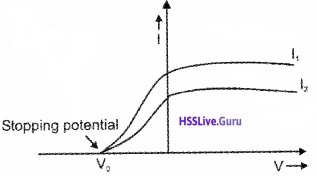As intensity increases photoelectric current also increases.

4. No. The work function of iron is very large.Question 6.
Lenard and Hallwachs investigated the phenomenon of photoelectric effect in details during 1886-1902 through experiments
1. Write any two characteristic features observed in the above experiment? (2)
2. Explain with reason

• Green light emit electron from certain metal surface while yellow light does not
• When the wavelength of incident light is decreased, the velocity of emitted photo electrons increases (2)

3. Complete the following statement about photoelectric effect.
The radiations having minimum frequency called…….falls on a metallic surcace, electrons are emitted from it. The metal which emits photoelectrons are called………The kinetic energy of photoelectrons emitted by a metal depends on………of the radiations, while intensity of the incident radiations depends on……… (1)
1. Any two statement of laws of photoelectric effect.

2. Explain with reason:

• Energy of incident photon is inversely proportional to its wavelength. Since λ of green light is less than that of yellow, it has larger energy. So it can emit photoelectrons
• As the wavelength decreases, frequency and hence energy of incident radiation increases and hence kinetic energy of photo electrons increases.

3. Threshold frequency, photosensitive frequency, number of photons.Question 7.
Figure below shows variation of stopping potential (V0) with frequency(?) of incident radiations for two different metals A and B.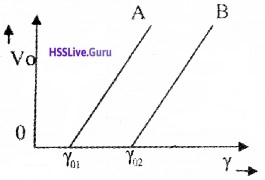1. Write down the values of work function A and B.

2. What is the significance of slope of the above graph? (1)

3. The value of stopping potential for A and B for a frequency γ01 (which is greaterthan γ02) of incident radiations are V1 and V0 respectively. Show that the slopes of the lines is equal to $$\frac{v_{1}-v_{2}}{\gamma_{01}-\gamma_{02}}$$. (3)
1. Work function of A, Φ01 – hν01
Work function of B, Φ01 – hν02.

2. The slope of the graph gives value of h/e.

3. For the metalA, hν1 = hν01 + eV1………..(1)
For the metal B, hν1 = hν02 + eV2…………..(2)
From equation (1) and (2)
01 + eV1 = hν02 + eV2
e(V1 – V2) = h(ν02 – ν01)
$$\frac{h}{e}=\frac{V_{1}-V_{2}}{v_{02}-v_{01}}$$Question 8.
Albert Einstein proposed a radically new picture of electromagnetic radiation to explain photoelectric effect.
1. Identify Einstein’s photoelectric equation? (1)
2. With the help of Einstein’s photoelectric equation explain the following facts.

• Kinetic energy of photoelectrons is directly proportional to frequency not on intensity.
• Existence of threshold frequency for a given photosensitive material. (2)

3. A metal whose work function is 2 eV is illuminated by light of wavelength 3 × 10-7m. Calculate

• threshold frequency
• maximum energy of photoelectrons
• the stopping potential. (3)

1. hν = hν0 + 1/2 mv2

2. Einstein’s photoelectric equation:
a. hν ∝ 1/2 mv2
1/2 mv2 ∝ ν
Hence kinetic energy is proportional to frequency.

b. hν – hν0 = 1/2 mv2
h(ν – ν0) = 1/2 mv2
ν should be greater than ν0 otherwise h(ν – ν0) is negative and is not possible.

3.a. Φ0 = hν0
ν0 = $$\frac{2 \times 1.6 \times 10^{-19}}{6.63 \times 10^{-34}}$$
= 4.8 × 1014 Hz

b. 1/2 mv2 = h(ν – ν0)
= 6.63 × 10-34 (1015 – 4.8 × 1014)
= 3.44 × 10-19J

c. ev0 = h(ν – ν0)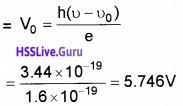Question 9.
The wave nature of electron was experimentally verified by diffraction of electron by Nickel crystal.

1. Name the experiment which establish wave nature of moving electron. (1)
2. With a neat diagram explain the existence of matter wave associated with an electron. (3)
3. An electron and a proton have same kinetic . energy which of these particles has shortest de-Broglie wave length? (2)

1. Davisson and Germer experiment.

2.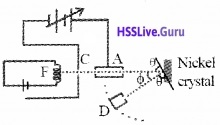Aim:
To confirm the wave nature of electron.
Experimental setup:
The Davisson and Germer Experiment consists of filament ‘F’, which is connected to a low tension battery. The Anode Plate (A) is used to accelerate the beam of electrons. A high voltage is applied in between A and C. ’N’ is a nickel crystal. D is an electron detector. It can be rotated on a circular scale. Detector produces current according to the intensity of incident beam.

Working:
The electron beam is produced by passing current through filament F. The electron beam is accelerated by applying a voltage in between A (anode) and C. The accelerated electron beam is made to fall on the nickel crystal.

The nickel crystal scatters the electron beam to different angles The crystal is fixed at an angle of Φ = 50° to the incident beam. The detector current for different values of the accelerating potential ‘V’ is measured. A graph between detector current and voltage (accelerating) is plotted. The shape of the graph is shown in figure.Analysis of graph:The graph shows that the detector current increases with accelerating voltage and attains maximum value at 54V and then decreases. The maximum value of current at 54 V is due to the constructive interference of scattered waves from nickel crystal (from different planes of crystal). Thus wave nature of electron is established.

Experimental wavelength of electron:
The wave length of the electron can be found from the formula
2d sinθ = n λ ………..(1)
From the figure, we get
θ + Φ + θ = 180°
2θ = 180 – Φ, 2θ = 180 – 50°
θ = 65°
for n = 1
equation (1) becomes
λ = 2d sinθ ………(2)
for Ni crystal, d = 0.91 A°
Substituting this in eq. (2), we get
wavelength λ = 1.65 A°Theoretical wave length of electron:
The accelerating voltage is 54 V
Energy of electron E = 54 × 1.6 × 10-19J
∴ Momentum of electron P = $$\sqrt{2 \mathrm{mE}}$$
P = $$\sqrt{2 \times 9.1 \times 10^{-31} \times 54 \times 1.6 \times 10^{-19}}$$
= 39.65 × 10-25 Kg ms-1
∴ De- Broglie wavelength λ = $$\frac{h}{P}$$The experimentally measured wavelength is found in agreement with de-Broglie wave length. Thus wave nature of electron is confirmed.

3.Mass of alpha particle is more than that of proton, hence it has shortest wavelength.Question 10.
In Geiger-Marsden Scattering experiment alpha particles of 5.5 MeV is allowed to fall on a thin gold foil of thickness 2.1 × 10-7m.
1. Draw Schematic diagram of above experimental arrangement.
2.In the above graph nearly 107 particles were detected when scattering angle is Zero. What do you understand by it?
3. Why gold foil is used in this experiment?
4. Does there exist any relation between impact parameter and scattering angle? If yes, explain your answer.
1.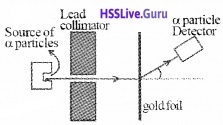2. Most of the alpha particles get unscattered means that most of the space in an atom is empty.

3. Atomic number of gold 79, so number of protons is very high. Hence scattering between alpha and nucleons is larger. Gold foil can be made very thin so that the alpha particles suffer not more than one scattering.

4. Yes.
As impact Parameter increases, scattering angle decreases.Question 11.
The study of emission line spectra of a material serve as a fingerprint for identification of the gas.

1. Name different series of lines observed in hydrogen spectrum. (1)
2. Draw energy level diagram of hydrogen atom? (2)
3. Write down the Balmer formula for wavelength of Hα line. (1)
4. Given Rydberg constant as 1.097 × 107m-1. Find the longest and shortest wavelength limit of Baler Series. (2)

1. Lyman series, Balmer series, Paschen series, Bracket series, Pfund series

2.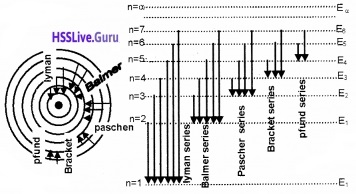3.4.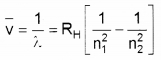Longest wavelength n1 = 2 and n2 = 3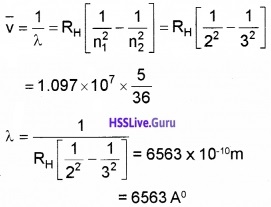Shortest Wavelength n1 = 2 and n2 = αQuestion 12.
Bohr combined classical and early quantum concept and gave his theory in the form of three postulates.

1. State three postulates of Bohr Model of atom? (2)
2. The total energy of an electron in ground state of hydrogen atom is -13.6eV. What is the significance of negative sign? (1)
3. The radius of innermost electron orbit of hydrogen atom is 5.3 × 1011m. What are the radii of n = 2 and n = 3 orbits? (2)

1. Bohr postulates:
Bohr combined classical and early quantum concepts and gave his theory in the form of three postulates.

• Electrons revolve round the positively charged nucleus in circular orbits.
• The electron which remains in a privileged path cannot radiate its energy.
• The orbital angular momentum of the electron is an integral multiple of h/2π.
• Emission or Absorption of energy takes place when an electron jumps from one orbit to an other.

2. Negative sign implies that the electrons are strongly bounded to the nucleus.

3.

• rn = n2a0 = 5.3 × 10-11m
• r1 = a0 = 5.3 × 10-11m
• r2 = 4a0 = 21.2 × 10-11m
• r3 = 9a0 = 47.7 × 10-11m.